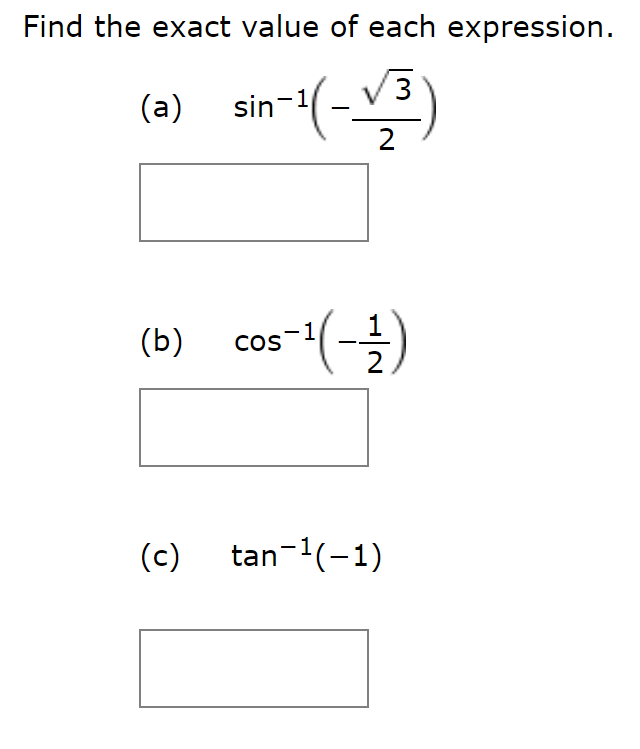# Find the exact value of each expression.sin 1(a)2cos(-)(b)COS2tan (1)(c)

Question
2 viewshelp_outlineImage TranscriptioncloseFind the exact value of each expression. sin 1 (a) 2 cos(-) (b) COS 2 tan (1) (c) fullscreen
check_circle

Step 1

we know that Range  of sine inverse function is[-pi/2,pi/2]. sin(pi/3)=sqrt3/2 and value of sine function is negative in fourth quadrant ,

Step 2

we know that Range  of cos inverse function is [0,pi]. cos(pi/3)=1/2 and value of cos function is negative in second quadrant ,

Step 3

we know that Range  of tan inverse function is(-pi/2,pi/2). tan(pi/4)...

### Want to see the full answer?

See Solution

#### Want to see this answer and more?

Solutions are written by subject experts who are available 24/7. Questions are typically answered within 1 hour.*

See Solution
*Response times may vary by subject and question.
Tagged in

### Other